Question

# Kris Company calculates its predetermined rates using practical volume, which is 325,000 units. The standard cost...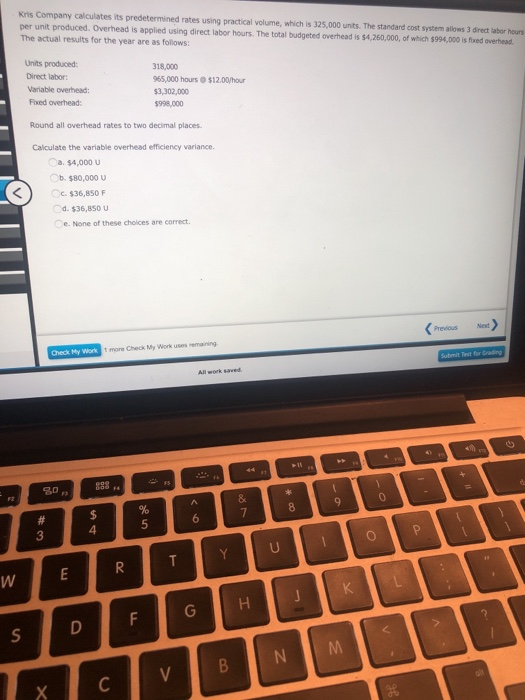Kris Company calculates its predetermined rates using practical volume, which is 325,000 units. The standard cost system allows 3 director hours per unit produced. Overhead is applied using direct labor hours. The total budgeted overhead is 14.260.000, of which 5994.000 is fed overhead The actual results for the year are as follows: Units produced: Direct labor: Variable overhead: Foxed overhead: 318,000 965,000 hours \$3,302,000 \$998,000 \$12.00/hour Round all overhead rates to two decimal places Calculate the variable overhead efficiency variance a \$4.000 U C. \$80,000 V c. \$36,850 F d. \$36,850 U e. None of these choices are correct. (previous Next Check My Work 1 more Check My Work uses remain Su Test for Grading 802 WELROUTPPY 's DFGHJK Ixc

= (SH - AH) * SR

= (318,000*3 - 965,000)*(4,260,000-994,000)/(325,000*3)

= (954,000 - 965,000)*3.35

= 36,850 U

#### Earn Coins

Coins can be redeemed for fabulous gifts.

Similar Homework Help Questions
• ### Kris Company calculates its predetermined rates using practical volume, which is 325,000 units. The standard cost...

Kris Company calculates its predetermined rates using practical volume, which is 325,000 units. The standard cost system allows 3 direct labor hours per unit produced. Overhead is applied using direct labor hours. The total budgeted overhead is \$4,260,000, of which \$994,000 is fixed overhead. The actual results for the year are as follows: Units produced: 318,000 Direct labor: 965,000 hours @ \$12.00/hour Variable overhead: \$3,302,000 Fixed overhead: \$998,000 Calculate the variable overhead spending variance. a.\$69,250 F b.\$69,250 U c.\$24,000 U...

• ### Kris Pty Ltd calculates its predetermined rates using practical volume, which is 325,000 units. The standard...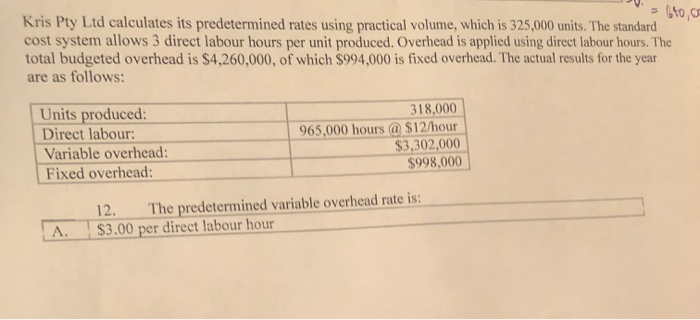Kris Pty Ltd calculates its predetermined rates using practical volume, which is 325,000 units. The standard cost system allows 3 direct labour hours per unit produced. Overhead is applied using direct labour hours. The total budgeted overhead is \$4,260,000, of which \$994,000 is fixed overhead. The actual results for the year are as follows: Units produced: Direct labour: Variable overhead: Fixed overhead: 318.000 965,000 hours @ \$12/hour \$3,302,000 \$998,000 12. The predetermined variable overhead rate is: \$3.00 per direct labour...

• ### Urbana Company calculates its predetermined manufacturing overhead rates using normal capacity, which is 288,000 units. The...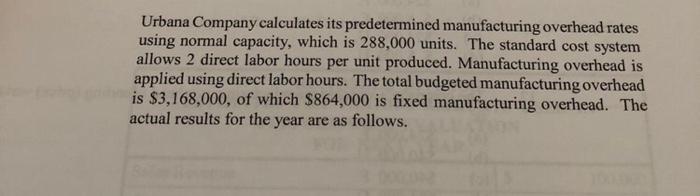Urbana Company calculates its predetermined manufacturing overhead rates using normal capacity, which is 288,000 units. The standard cost system allows 2 direct labor hours per unit produced. Manufacturing overhead is applied using direct labor hours. The total budgeted manufacturing overhead is \$3,168,000, of which \$864,000 is fixed manufacturing overhead. The actual results for the year are as follows. 5. The company's variable manufacturing overhead efficiency (quantity) variance is: \$24,000 F. (b) \$40,000 U. \$40,000 F. \$24,000 U. (a) URBANA COMPANY...

• ### Use the following information to answer Questions 9 through 13. Urbana Company calculates its predetermined manufacturing...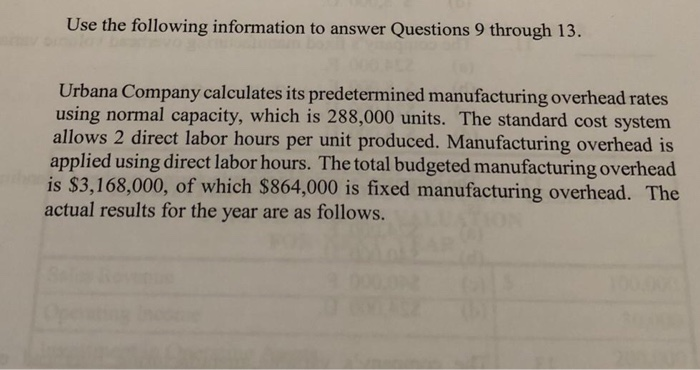Use the following information to answer Questions 9 through 13. Urbana Company calculates its predetermined manufacturing overhead rates using normal capacity, which is 288,000 units. The standard cost system allows 2 direct labor hours per unit produced. Manufacturing overhead is applied using direct labor hours. The total budgeted manufacturing overhead is \$3,168,000, of which \$864,000 is fixed manufacturing overhead. The actual results for the year are as follows. URBANA COMPANY ACTUAL RESULTS FOR PRODUCTION FOR CURRENT YEAR 280,000 Units produced...

• ### Question text Lance Production Company has the following information: Standard fixed factory overhead rates per direct...

Question text Lance Production Company has the following information: Standard fixed factory overhead rates per direct labor-hour \$1.50 Standard variable factory overhead rates per direct labor-hour \$5.00 Actual number of units produced 6,000 units Actual factory overhead costs (includes \$70,000 fixed) \$78,000 Actual direct labor hours 6,000 hours Standard factory overhead rates are based on a normal monthly volume of 5,000 units (1 standard direct labor-hour per unit). What is Lance's variable overhead efficiency variance? Select one: A. \$3,000 (F)...

• ### eBook Calculator Applied Overhead and Unit Overhead Cost: Plantwide Rates Seco, Inc., produces two types of...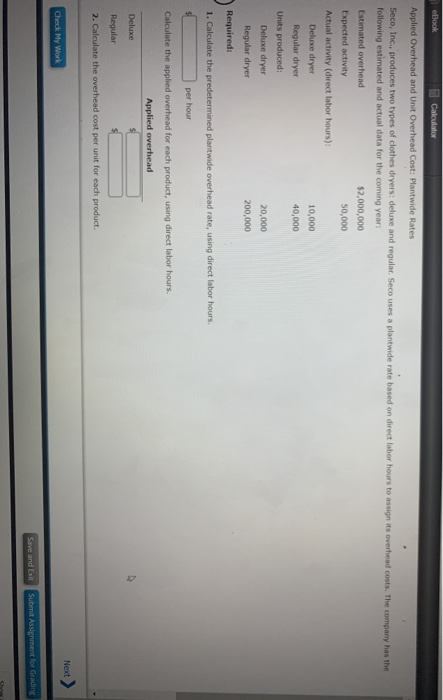eBook Calculator Applied Overhead and Unit Overhead Cost: Plantwide Rates Seco, Inc., produces two types of clothes dryers: deluxe and regular. Seco uses a plantwide rate based on direct labor hours to assign its overhead costs. The company has the following estimated and actual data for the coming year Estimated overhead \$2,000,000 Expected activity 50,000 Actual activity (direct labor hours): Deluxe dryer 10,000 Regular dryer 40,000 Units produced Deluxe dryer 20,000 Regular dryer 200,000 Required: 1. Calculate the predetermined plantwide...

• ### Judd Company uses standard costs for its manufacturing division. Standards specify 0.1 direct labor hours per...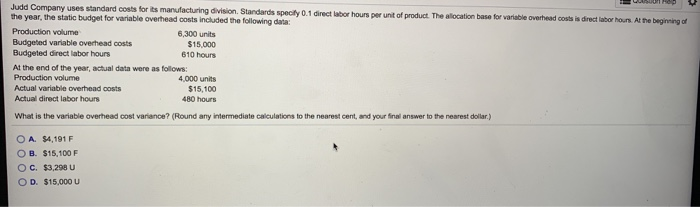Judd Company uses standard costs for its manufacturing division. Standards specify 0.1 direct labor hours per unit of product. The allocation base for variable overhead costs is direct labor hours. At the beginning of the year, the static budget for variable overhead costs included the following data: Production volume 6,300 units Budgeted variable overhead costs \$15,000 Budgeted direct labor hours 610 hours At the end of the year, actual data were as follows: Production volume 4.000 units Actual variable overhead...

• ### Flandro Company uses a standard cost system and sets its predetermined overhead rate on the basis...

Flandro Company uses a standard cost system and sets its predetermined overhead rate on the basis of direct labor-hours. The following data are taken from the company’s planning budget for the current year: Denominator activity (direct labor-hours) 16,000 Variable manufacturing overhead cost \$ 55,200 Fixed manufacturing overhead cost \$ 146,400 The standard cost card for the company’s only product is given below: Inputs (1) Standard Quantity or Hours (2) Standard Price or Rate Standard Cost (1) × (2) Direct materials...

• ### In March, Leona Company made 4,500 units of its product. Leona uses a standard cost system...

In March, Leona Company made 4,500 units of its product. Leona uses a standard cost system and applies overhead cost based on direct labor hours.   Standard: DLH per unit 2.50 Variable overhead per DLH \$1.75 Fixed overhead per DLH \$3.10 Budgeted variable overhead \$21,875 Budgeted fixed overhead \$38,750 Actual: Direct labor hours 10,000 Variable overhead \$26,250 Fixed overhead \$38,000 Refer to LeonaCompany. Using the four-variance approach, what is the fixed overhead spending variance? a. \$7,000.00 U b. \$ 750.00 F...

• ### Concita Ltd. uses a standard cost system and sets predetermined overhead rates on the basis of...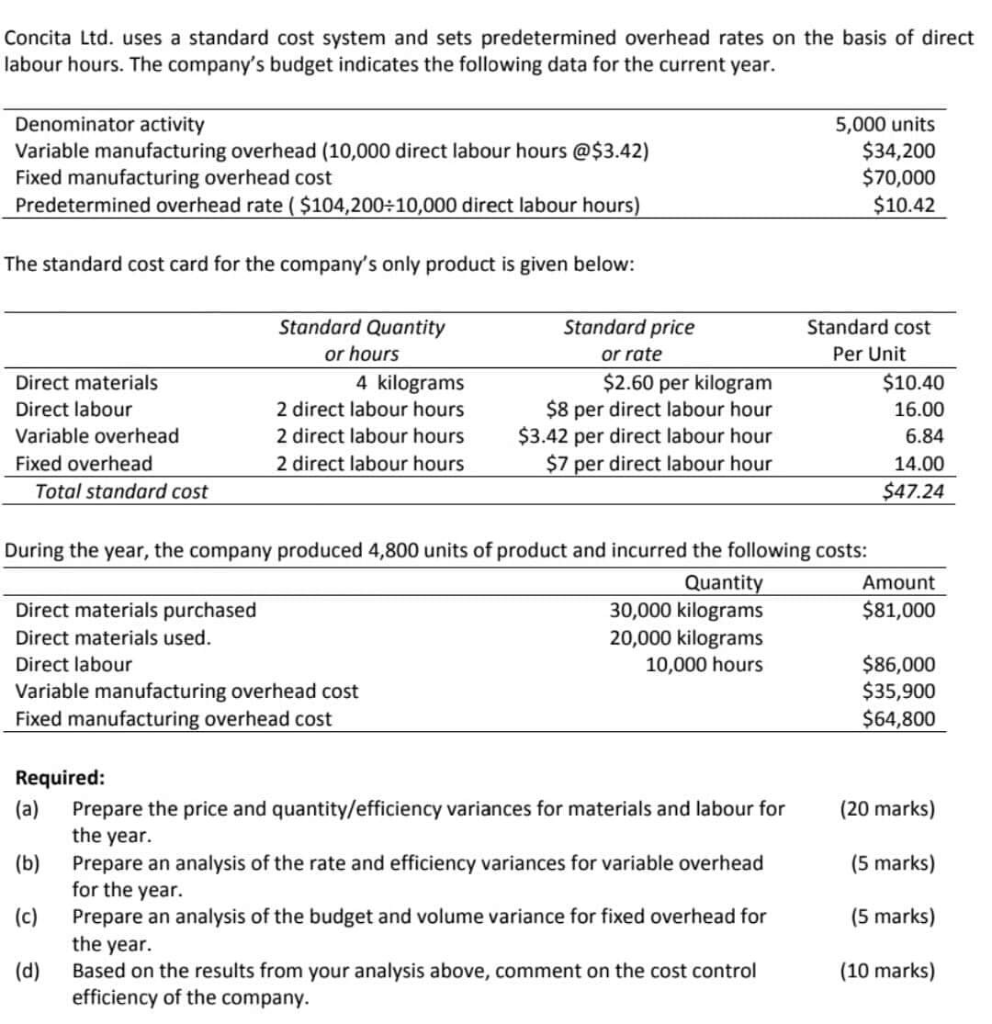Concita Ltd. uses a standard cost system and sets predetermined overhead rates on the basis of direct labour hours. The company's budget indicates the following data for the current year. Denominator activity Variable manufacturing overhead (10,000 direct labour hours @\$3.42) Fixed manufacturing overhead cost Predetermined overhead rate (\$104,200 10,000 direct labour hours) 5,000 units \$34,200 \$70,000 \$10.42 The standard cost card for the company's only product is given below: Standard Quantity Standard price Standard cost or hours Per Unit or...